Search IntMath
Close

450+ Math Lessons written by Math Professors and Teachers

5 Million+ Students Helped Each Year

1200+ Articles Written by Math Educators and Enthusiasts

Simplifying and Teaching Math for Over 23 Years

# 5. Integration: Other Trigonometric Forms

by M. Bourne

We can use the trigonometric identities that we learned earlier to simplify the integration process.

The main identities are shown here for reference:

cos^2x+sin^2x=1

1+tan^2x=sec^2x

1+cot^2x=csc^2x

2\ cos^2x=1+cos 2x

2\ sin^2x=1-cos 2x

The process that we use involves using the trigonometric ratios to simplify the expression, or to get the expression into a form that can be integrated.

## Integrating a Product of Powers of Sine and Cosine - one power odd

To integrate a product of powers of sine and cosine, we use

cos^2x+sin^2x=1

if at least one of the powers is odd.

### Example 1

Integrate: int3\ cos^3x\ dx.

int3\ cos^3x\ dx

=3int(cos^2x)cos x\ dx

=3int(1-sin^2x)cos x\ dx

=3int(cos x\ -sin^2x\ cos x\) dx

Letting u=sin x, and thus du=cos x\ dx gives:

=3[sin x+K_1-intu^2du]

=3[sin x-(u^3)/3+K]

=3[sin x-(sin^3x)/3+K]

## Integrating a Product of Powers of Sine and Cosine - powers even

We use

2\ cos^2x=1+cos 2x

or

2\ sin^2x=1-cos 2x

if the power of sin x or cos x is even.

### Example 2

Integrate: intcos^2\ 2x\ dx.

intcos^2 2x\ dx

Let u=2x, then du=2\ dx

This gives:

intcos^2u(du)/2 =1/2intcos^2 u\ du

=1/2int(1+cos 2u)/2du

=1/4int1+cos 2u\ du

=1/4[u+(sin 2u)/2]+K

=1/4[2x+(sin 2(2x))/2]+K

=x/2+(sin 4x)/8+K

### Example 3

Integrate: 6intcot^3x\ dx.

We have:

6intcot^3x\ dx =6int(cot^2 x)cot x\ dx

=6int(csc^2x-1)cot x\ dx

=6(intcsc^2 x\ cot x\ dx {:-intcot x\ dx)

Take the intcsc^2x\ cot x\ dx integral first:

Let u = cot x, then du = -csc^2x\ dx

So

intcsc^2x\ cot x\ dx =-intu\ du

=-u^2/2+C

=-(cot^2x)/2+C

For the second integral, from our table, we have

intcot x\ dx=ln\ |sin x|+C

Returning to the main question:

6intcot^3x\ dx =6(intcsc^2x\ cot x\ dx- {:intcot x\ dx)

=6(-(cot^2x)/2-ln\ |sin x|)+K

=-3\ cot^2x-6\ ln\ |sin x|+K

## Application - Root Mean Square Value

The root mean square value of the function y with respect to x is given by:

y_("rms")=sqrt(1/T int_0^T y^2dx

where T is the period of y.

### Effective Current

A common use of this concept is effective current. This is the value of the direct current that would produce the same quantity of heat energy in the same time as a certain alternating current. It is used in the design of heaters.

### Example 4

Find the root mean square (rms) value of i = 3 + 2 cos t.

In this case, T = 2π.

So

i_("rms") =sqrt(1/Tint_0^Ti^2dt)

=sqrt(1/(2pi)int_0^(2pi)(3+2\ cos t)^2dt)

Now

(3+2\ cos t)^2=9+12\ cos t+4\ cos^2 t

Since cos 2t = 2 cos^2 t - 1, it follows that cos^2t=(cos 2t+1)/2.

So

(3+2\ cos t)^2 =9+12 cos t+4((cos 2t+1)/2)

=9+12 cos t+2(cos 2t+1)

=11+12\ cos t+2\ cos 2t

So

int_0^Ti^2dt =int_0^(2pi)(11+12\ cos t+2\ cos 2t)dt

=[11t+12\ sin t+sin 2t]_0^(2pi)

=22pi

And, finally:

i_("rms") =sqrt(1/Tint_0^Ti^2dt)

=sqrt((22pi)/(2pi))

=sqrt11

=3.317

This is a graph of our cosine current with the RMS effective current shown.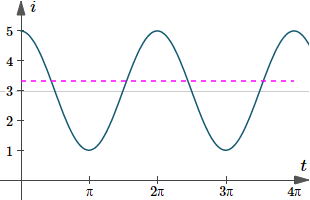Graph of i(t)=3+2cos(t), with the RMS current indicated by the dashed magenta line.

### Example 5

For a current i given by i = i0 sin ωt, show that the root-mean-square of the current for one period is (i_0)/sqrt2.

In this case,

T=(2pi)/omega

So

i_("rms")=sqrt(1/T int_0^Ti^2dt)

=sqrt(omega/(2pi)int_0^((2pi)/omega)(i_0\ sin omegat)^2dt)

=sqrt((omega(i _0)^2)/(2pi)int_0^((2pi)/omega)sin^2omegat\ dt)

Let's just take the integral part first, and put

sin^2omegat=(1-cos 2 omegat)/2

int_0^(2pi//omega)sin^2omegat\ dt =1/2int_0^(2pi//omega)(1-cos 2 omegat)dt

=1/2[t-(sin 2omega t)/(2omega)]_0^(2pi//omega)

=1/2[((2pi)/omega-0)-(0-0)]

=pi/omega

So

i_("rms")=sqrt((omega(i_0)^2)/(2pi)int_0^((2pi)/omega)sin^2omegat\ dt)

=sqrt{(omega (i_0)^2)/(2pi)pi/omega}

=sqrt(((i_0)^2)/2)

=(i_0)/sqrt2

This is what the question required us to show.

## Exercises

Integrate each of the given functions:

1. int_(pi//3)^(pi//2)sqrt(cos x)\ sin^3x\ dx

int_(pi//3)^(pi//2)sqrt(cos x)\ sin^3x\ dx

Write sin^3x  = sin^2x\ sin x  = (1 - cos^2x)(sin x)

So, taking the indefinite case first:

sqrt(cos x) sin^3x

=cos^(1//2)x(1-cos^2x)(sin x)

=(cos^(1//2)x-cos^(5//2)x)(sin x)

Put u = cos x then du = -sin x\ dx

So

intsqrt(cos x)\ sin^3x\ dx =int(cos^(1//2)x-cos^(5//2)x)(sin x)dx

=-int(u^(1//2)-u^(5//2))du

=-(2u^(3//2))/3+(2u^(7//2))/7+K

=-(2\ cos^(3//2) x)/3+(2\ cos^(7//2)x)/7+K

So we have for the definite case:

int_(pi//3)^(pi//2)sqrt(cos x)\ sin^3x\ dx =[-(2 cos^(3//2)x)/3+(2 cos^(7//2)x)/7]_(pi//3)^(pi//2)

=[(0+0)-(-0.23570+0.02525)]

=0.2104

The solution for Exercise 1 represents the area under the curve y(x)=sqrt(cos x)\ sin^3x\ dx between pi/3 <= x <= pi/2. Here is that graph: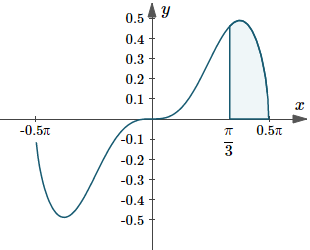Graph of y(x)=sqrt(cos x)\ sin^3x, indicating the area under the curve from x=pi/3 to x=pi/2.

Zooming out that graph shows it's periodic (it repeats itself) with period 2pi. There are holes in the graph because of the sqrt(cos x) part (we can't have the square root of a negative number).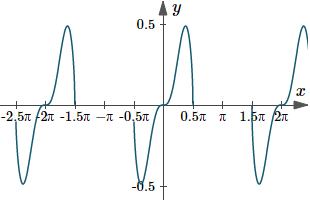Graph of y(x)=sqrt(cos x)\ sin^3x dx, zoomed out to see its periodic nature.

2. int_0^1sin^2 4x\ dx

int_0^1sin^2 4x\ dx

We recall that

2\ sin^2theta=1-cos 2theta

In this case, if θ = 4x,

2 sin^2 4x =1-cos [2(4x)] =1-cos 8x

So sin^2 4x=(1-cos 8x)/2

int_0^1sin^2 4x dx =1/2int_0^1(1-cos 8x) dx

=1/2[x-(sin 8x)/8]_0^1

=1/2[(1-0.9894/8)-(0-0)]

=0.4382

Here is the graph of the integration we just found, indicating the area under the curve y=sin^2 4x.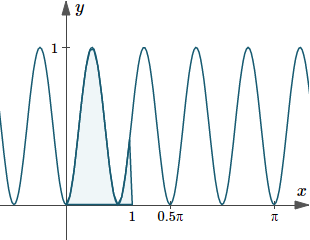Graph of y(x)=sin^2 4x, indicating the area under the curve from x=0 to x=1.

3. intcot 4x\ csc^4 4x\ dx

int cot 4x\ csc^4 4x\ dx

We write the expression under the integral sign as follows:

cot 4x\ csc^4 4x\ dx =(csc^3 4x)\ cot 4x\ csc\ 4x

Then, let u = csc\ 4x and so we have du = -4\ csc\ 4x\ cot 4x\ dx

That is, -(du)/4 = csc\ 4x\ cot 4x\ dx

Now we can perform the integral:

int cot 4x\ csc^4 4x\ dx =int(csc^3 4x)\ cot 4x\ csc\ 4x\ dx

=-1/4intu^3du

=-u^4/16+K

=-(csc^4 4x)/16+K

4. intsqrt(tan x)\ sec^4x\ dx

Recall that

sec^2x=1+tan^2x

So we can write the part under the integral as:

sqrt(tan x)\ sec^4 x =(tan^(1//2) x)\ sec^2 x\ sec^2 x

=(tan^(1//2) x )(1+tan^2 x )sec^2 x

=(tan^(1//2) x+tan^(5//2)x)sec^2 x

Next, we put u = tan x, giving du = sec^2x\ dx

So our integral becomes:

int (tan^(1//2) x+tan^(5//2)x)sec^2 x\ dx =int(u^(1//2)+u^(5//2))du

=2/3u^(3//2)+2/7u^(7//2)+K

=2/3tan^(3//2)x+2/7tan^(7//2)x+K

5. int_(pi//6)^(pi//3)(2dx)/(1+sin x

int_(pi//6)^(pi//3)(2\ dx)/(1+sin x)

We need to re-express this and we make use of a fairly common technique. We multiply the numerator (top) and denominator (bottom) of the fraction by the conjugate of the denominator. (The conjugate has the opposite sign in the middle. In this case, it's a minus sign.)

We will make use of this important result:

sin^2 x+ cos^2 x = 1

1/(1+sin x) =1/(1+sin x)xx(1-sin x)/(1-sin x)

=(1-sin x)/(1-sin^2x)

=(1-sin x)/(cos^2 x)

=sec^2x-(sin x)/(cos^2 x)

Now we can integrate.

Putting u = cos x in the right hand part, we have:

du = -sin x\ dx

So

int_(pi//6)^(pi//3)(2\ dx)/(1+sin x)

=2int_(pi//6)^(pi//3)(sec^2x-(sin x)/(cos^2x))dx

=2[tan x-1/(cos x)]_(pi//6)^(pi//3)

=2[(1.73215-2)- {:(0.57735-1.15470)]

=0.6190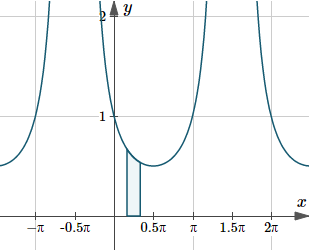Graph of y(x)=2/(1+sin(x)), indicating the area under the curve from x=pi/6 to x=pi/3.

### Application - Length of a Curve

The length s of the arc of a curve y = f(x) from x = a to x = b is given by:

s=int_a^bsqrt(1+((dy)/dx)^2dx

Find the length of the curve y = ln (cos x) from x=0 to x=pi/3.

The curve in this problem is y = ln cos x.

We need its derivative:

(dy)/(dx)=-(sin x)/(cos x)=-tan x

For this problem, we'll make use of an earlier result,

1+tan^2 x=sec^2 x

Applying the formula gives:

s=int_a^bsqrt(1+((dy)/dx)^2)dx

=int_0^(pi//3)sqrt(1+(-tan x)^2)dx

=int_0^(pi//3)sqrt(1+tan^2x) dx

=int_0^(pi//3)sqrt(sec^2x) dx

=int_0^(pi//3)sec x\ dx

=[ln\ |sec x+tan x|]_0^(pi//3)

=[ln\ |sec (pi/3)+tan (pi/3)| {:-|ln(1)+ln(0)|]

=1.317

Here's the graph of the arc length we just found (in pink). I needed to take the absolute value of the cos(x) values, otherwise there would be gaps in the graph (whenever cos(x) was negative).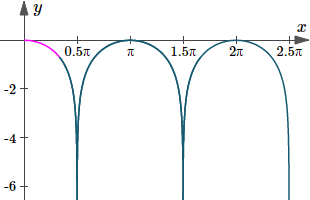Graph of y(x)=ln|cos x|, with the curve length we just found indicated in magenta (pink).

## Problem SolverThis tool combines the power of mathematical computation engine that excels at solving mathematical formulas with the power of GPT large language models to parse and generate natural language. This creates math problem solver thats more accurate than ChatGPT, more flexible than a calculator, and faster answers than a human tutor. Learn More.